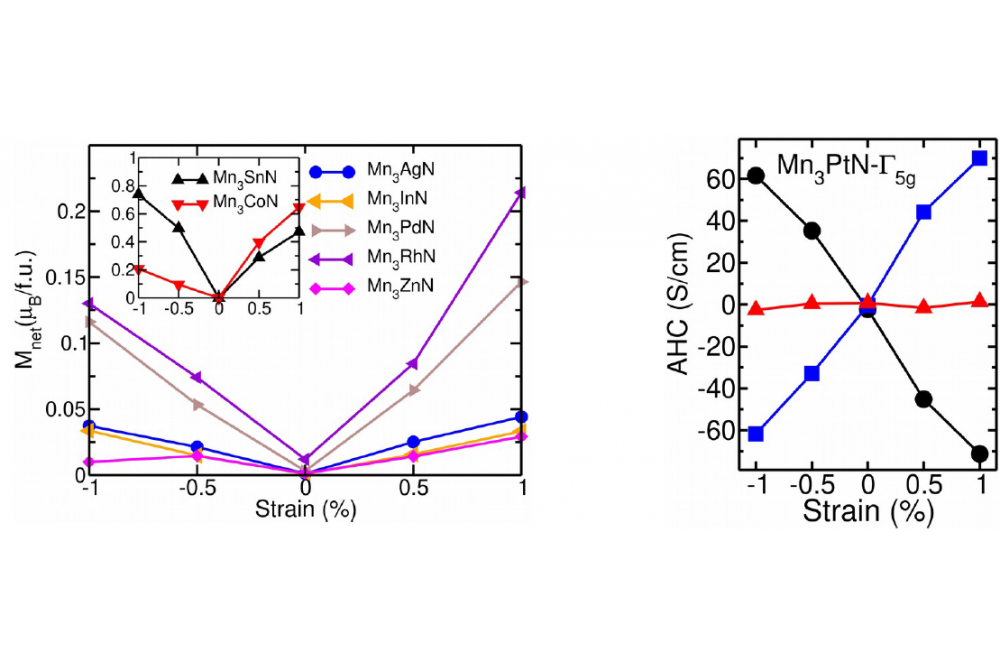High Throughput Screening for Spin-Polarized Current in Noncollinear MaterialsSingh, Harish Kumar_High Throughput Screening for Spin-Polarized Current in Noncollinear Materials_Figure1

Figure:1. The net magnetization induced by piezomagnetic effect for the Mn-based APVs (left). The calculated AHC of Mn3PtN. The circle, square, and triangle correspond to the σx, σy, and σz AHC tensor, respectively. The negative (positive) strain corresponds to the compressive (tensile) biaxial strain.

Introduction

Like perovskite materials, antiperovskites (APVs) display many intriguing physical properties. Particularly, the magnetic APVs with noncollinear (NC) antiferromagnets exhibit properties like magnetocaloric, barocaloric, piezomagmetic effect (PME), anomalous Hall conductivity (AHC), and anomalous Nernst conductivity (ANC). This class of materials could show various potential applications in the future. In this work, we carried out a systematic analysis on 54 cubic APV systems (Pm3m) with chemical formula M3XZ (M = Cr, Mn, Fe, Co and Ni, Z= C and N, X=Li-Bi except for noble gases and rare earth materials.) to find out the lowest energy magnetic configuration by analyzing the energetic of three magnetic phases (Γ4g, Γ5g, and FM). For APVs with NC ground state, the PME AHC, and ANC was determined for the cubic phase and tetragonal phase (obtained by imposing compressive and tensile biaxial strain).

Methods

The density functional theory (DFT) calculations were performed using the projector augmented wave (PAW) method as effectuated in the VASP package. The AHC was evaluated using the WannierTools code, where the required accurate tight-binding models are obtained by the maximally localized Wannier functions (MLWFs) using the Wannier90 code.

Results

We found 16 antiferromagnetic APVs with NC magnetic ground state. The magnetic ground states of reported APVs are in good agreement with experimental measurements. At 1.0% compressive strain, a large PME of 0.73 μB/f.u. is observed for the MnB/f.u. is observed for the Mn3SnN (see Figure 1). The phase transition between different magnetic ordering (Γ4g and Γ5g) is realized by strain. Like, Cr3SnN has Γ4g as the lowest energy magnetic ordering without strain and with compressive strain. However, the magnetic ordering transforms to Γ5g state on applying tensile strain. For cubic structure, the APVs Mn3XN (X = Ag, Au, Ga, Hg, In, Pt, Sn, and Zn) exhibit zero AHC in Γ5g state. The Cr3SnN exhibits AHC of 278 S/cm in Γ4g state. Similarly, the other APVs show finite AHC in Γ4g state. At 1% tensile strain, the maximum AHC of 693 S/cm observed for the σz component of Cr3IrN. From our calculations, the ANC of Mn3NiN is as large as 16569 S/cm.eV.

Discussion

Based on our PME analysis, we propose Cr- and Mn-based NC systems with large PME as potentials candidates for magnetoelectric composite. Switching to different magnetic phases will change the properties of the materials significantly. The magnetic symmetry allows the finite AHC/ANC only in Γ4g configuration of the cubic phase, whereas the finite AHC and ANC are possible for both Γ4g and Γ5g configurations with biaxial strain. The tuning of AHC/ANC is achievable with strain, which can be ascribed to the Weyl points in the proximity of the Fermi energy. Realizing such an effect could be interesting for the potential AFM spintronic applications.

Last Update

• Last Update: 2020-07-17 14:13